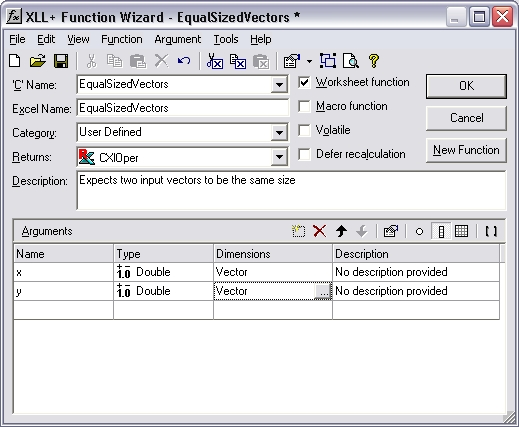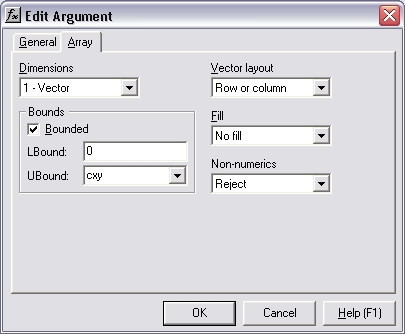# HOW TO: How can I make sure that two vector inputs are the same size?

Reference: Q0036

• XLL+ for Visual Studio 2005 - 5.0
• XLL+ for Visual Studio .NET - 4.2, 4.3.1, 5.0
• XLL+ for Visual Studio 6 - 3, 4.1, 4.2, 4.3.1, 5.0

## HOW TO: How can I make sure that two vector inputs are the same size?

### Question

My add-in function expects two vector inputs to be the same size. Can I use the Function Wizard to specify this constraint?

You can modify your arguments to be bounded input arrays. The XLL+ Function Wizard has a tab which lets you set the bounds of an array, so that they are tied to a named variable (or to a constant). The code which is generated will automatically check that all matrix and vector inputs satisfy the constraints you apply.

By using the same named variable for each input, you can ensure that each input is the same size.

If the second input is not of the expected size, then an error value is returned, e.g.:

`#Error: expected 5 items in y`

### Example

Problem: constrain two vector inputs to be the same size.

#### Steps

1. Create a new function, `EqualSizedVectors()`, using the XLL+ Function Wizard. It takes two arguments `x` and `y`, each of which is defined as a vector of doubles.2. Select the Dimensions column of the row containing the definition of `x`, and click on the `...` button. (Or use the short-cut Ctrl+E.) This will display the Edit Argument dialog.

Select the Array tab.

In the Bounds box, check the Bounded check-box and set the upper bound (UBound) to be cxy, as shown below.3. Press OK to close the dialog.
4. Select the `y` argument, open the Edit Argument dialog, and make exactly the same changes as you did for `x`.

5. Press OK to close the dialog.
6. Inspect the changes to the Function Wizard. The Dimensions column now contains the new definitions of `x` and `y`: `Vector (0 to cxy)`.7. Save the function.

#### Code

The code generated is shown below:

```// Function:    EqualSizedVectors
// Purpose:     Expects two input vectors to be the same size

//{{XLP_SRC(EqualSizedVectors)
// NOTE - the FunctionWizard will add and remove mapping code here.
//    DO NOT EDIT what you see in these blocks of generated code!
IMPLEMENT_XLLFN2(EqualSizedVectors, "RPP", "EqualSizedVectors",
"x,y", "User Defined", "Expects two input vectors to be the"
" same size", "No description provided\000No description"
" provided\000", "B0(0,cxy)x No description provided\0B0(0"
",cxy)y No description provided\0appscope=1\0", 1)

extern "C" __declspec( dllexport )
LPXLOPER EqualSizedVectors(const COper* x, const COper* y)
{
XLL_FIX_STATE;
CXlOper xloResult;
BOOL bOk = TRUE;
long cxy = -1;
std::vector<double> vecx;
bOk = bOk && x->ReadVectorBounded(vecx, "x", xloResult, 0, cxy);
std::vector<double> vecy;
bOk = bOk && y->ReadVectorBounded(vecy, "y", xloResult, 0, cxy);
if (!bOk)
return xloResult.Ret();
//}}XLP_SRC

// TODO - Set the value of xloResult
return xloResult.Ret();
}```

#### Explanation

The interesting code is shown in bold above.

1. The bounds variable `cxy`, which we entered as UBound in the Edit Argument dialog, is declared, and is initialized to `-1`. This value represents "not yet known".
2. A reference to `cxy` is passed to COper::ReadVectorBounded when reading both x and y.
3. The first time ReadVectorBounded receives `cxy`, it contains -1 (meaning "not yet known"), and ReadVectorBounded simply sets its value to the size of `x`.
4. On the second call to ReadVectorBounded `cxy` contains a non-negative integer, and is therefore treated as a fixed size constraint. If ``` y``` does not contain `cxy` cells then an error is returned.

#### Result

If, for example, `x` contains 6 cells and `y` contains 4 cells, then the following error value is returned

`#Error: expected 6 items in y`

and the function does not continue.